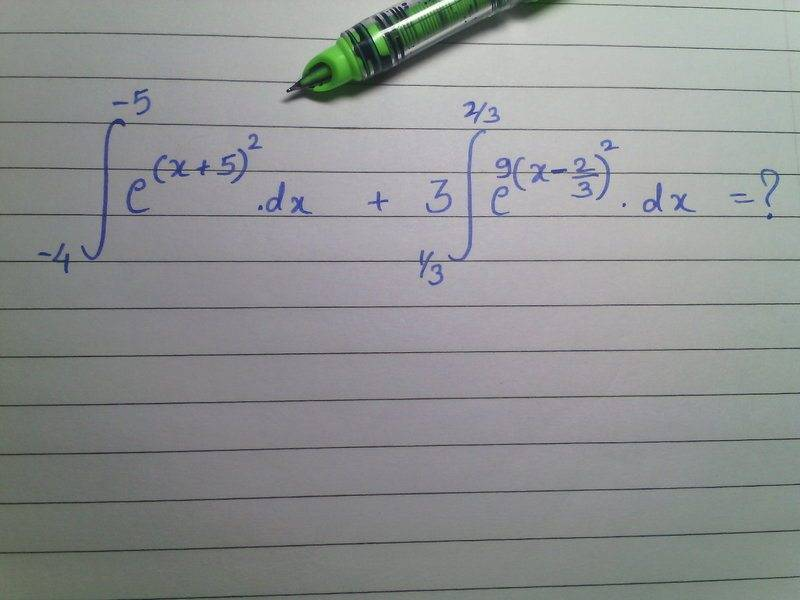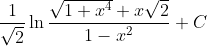# Really hard integrals?

royjsky
Hard ,but famous and bautiful :

$$\int_{0}^{\infty}sin(x^2)dx$$

I believe this uses multivariable calculus since I recall you must first prove the definite integral of the e^-x^2 which requires double integrals and a change of variables.

royjsky
I'm just out of Calc 1, so I'm not sure if I even have the knowledge to solve this... but here's where I am now. I can't tell if I complicated it even more, or if I'm closer to getting the solution.

$$\int (r-1)^{1/5}ln(r) - r^{-1} (r-1)^{1/5} dr$$

I used u-substitution (well, r-substitution), where $$r = x^5 + 1$$. After the substitution I used integration by parts, and now I'm unsure if that was even the right path. Please let me know!

And please tell us how to do $$\int_{0}^{\infty}sin(x^2)dx$$
I think you need multivariable to do that.

Corbeau
Here is a hard nut to crack. Been trying to solve it for a while, can't confirm it's actually solvable.

1/ ( x ( ln (x+1) -1 ) )

suprabhe
x^2arctan(5x)

Gold Member
Last edited by a moderator:
•ComplexVar89
MAGNIBORO
$$\int_{0}^{\pi}\frac{cos(nx)-cos(na)}{cos(x)-cos(a)} dx$$

and

$$\int_{0}^{\infty }\frac{x}{e^{x}-1} dx$$

Last edited:
Try this.Ritam Dutta
Hard ,but famous and bautiful :

$$\int_{0}^{\infty}sin(x^2)dx$$
it should come from common sense i think. seems the analytical method is going to be just WOOW

Gold Member
I was just thinking this thread is quite reminiscent of that book (without Nahin's witty commentary, of course.)

•Demystifier
quarantine2020
This is my answer, tell me if i did something wrong :).this is a “simpler” result I got from unraveling your solution & re-packaging it. I want to figure out how to get it in this form in a more natural way. As of now I’m stuck. Right now I’m working with a Pythagorean triangle
Opposite = x*sqrt(2)
Hypotenuse = sqrt(1+x^4)

and the solution is 1/sqrt(2)*ln(sec(angle)+tan(angle)) + C

I see some kind of pattern here but it’s a little opaque. Any way to clear this up & produce a really elegant solution?

Gold Member
Try:

$$\int_{0}^{1} e^{-x^{x}} dx$$

Ssnow

akil
try the integral of sin(lnx) by using eulers formula

Aritro Biswas
Try $$\int{\frac{(1+x^{2})dx}{(1-x^{2})\sqrt{1+x^{4}}}}$$
$$\frac{1}{2\sqrt{2}}\ln \left| { \frac{\sqrt{2}+{\sqrt{x^2+\frac{1}{x^2}}}} {\sqrt{2}-{\sqrt{x^2+\frac{1}{x^2}}}}} \right|$$